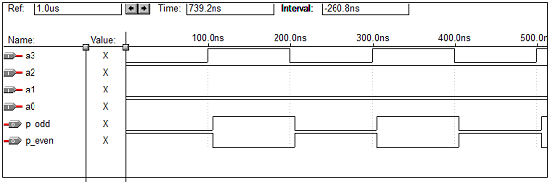# VHDL Programming Combinational Circuits

This chapter explains the VHDL programming for Combinational Circuits.

## VHDL Code for a Half-Adder

VHDL Code:

Library ieee;
use ieee.std_logic_1164.all;

port(a,b:in bit; sum,carry:out bit);

begin
sum<= a xor b;
carry <= a and b;
end data;


### Waveforms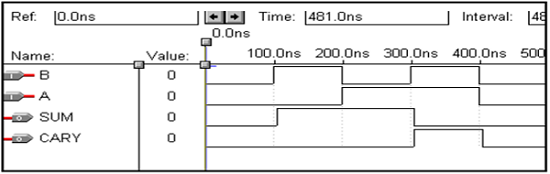## VHDL Code for a Full Adder

Library ieee;
use ieee.std_logic_1164.all;

entity full_adder is port(a,b,c:in bit; sum,carry:out bit);

begin
sum<= a xor b xor c;
carry <= ((a and b) or (b and c) or (a and c));
end data;


### Waveforms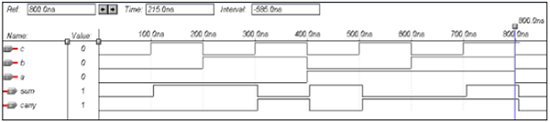## VHDL Code for a Half-Subtractor

Library ieee;
use ieee.std_logic_1164.all;

entity half_sub is
port(a,c:in bit; d,b:out bit);
end half_sub;

architecture data of half_sub is
begin
d<= a xor c;
b<= (a and (not c));
end data;


### Waveforms## VHDL Code for a Full Subtractor

Library ieee;
use ieee.std_logic_1164.all;

entity full_sub is
port(a,b,c:in bit; sub,borrow:out bit);
end full_sub;

architecture data of full_sub is
begin
sub<= a xor b xor c;
borrow <= ((b xor c) and (not a)) or (b and c);
end data;


### Waveforms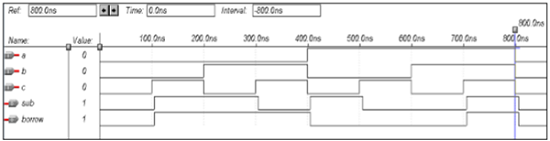## VHDL Code for a Multiplexer

Library ieee;
use ieee.std_logic_1164.all;

entity mux is
port(S1,S0,D0,D1,D2,D3:in bit; Y:out bit);
end mux;

architecture data of mux is
begin
Y<= (not S0 and not S1 and D0) or
(S0 and not S1 and D1) or
(not S0 and S1 and D2) or
(S0 and S1 and D3);
end data;


### Waveforms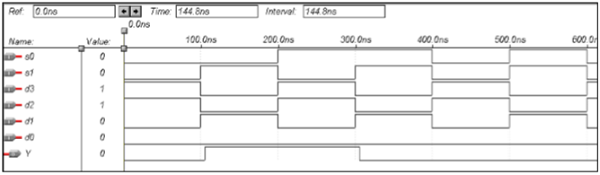## VHDL Code for a Demultiplexer

Library ieee;
use ieee.std_logic_1164.all;

entity demux is
port(S1,S0,D:in bit; Y0,Y1,Y2,Y3:out bit);
end demux;

architecture data of demux is
begin
Y0<=  ((Not S0) and (Not S1) and D);
Y1<=  ((Not S0) and S1 and D);
Y2<=  (S0 and (Not S1) and D);
Y3<=  (S0 and S1 and D);
end data;


### Waveforms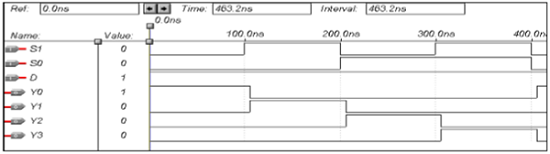## VHDL Code for a 8 x 3 Encoder

library ieee;
use ieee.std_logic_1164.all;

entity enc is
port(i0,i1,i2,i3,i4,i5,i6,i7:in bit; o0,o1,o2: out bit);
end enc;

architecture vcgandhi of enc is
begin
o0<=i4 or i5 or i6 or i7;
o1<=i2 or i3 or i6 or i7;
o2<=i1 or i3 or i5 or i7;
end vcgandhi;


### Waveforms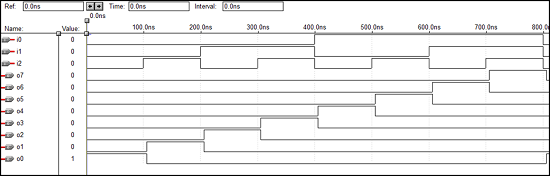## VHDL Code for a 3 x 8 Decoder

library ieee;
use ieee.std_logic_1164.all;

entity dec is
port(i0,i1,i2:in bit; o0,o1,o2,o3,o4,o5,o6,o7: out bit);
end dec;

architecture vcgandhi of dec is
begin
o0<=(not i0) and (not i1) and (not i2);
o1<=(not i0) and (not i1) and i2;
o2<=(not i0) and i1 and (not i2);
o3<=(not i0) and i1 and i2;
o4<=i0 and (not i1) and (not i2);
o5<=i0 and (not i1) and i2;
o6<=i0 and i1 and (not i2);
o7<=i0 and i1 and i2;
end vcgandhi;


### Waveforms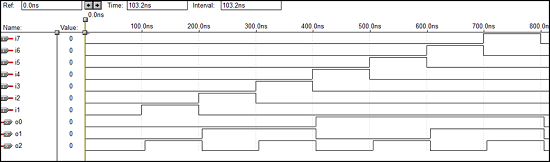## VHDL Code – 4 bit Parallel adder

library IEEE;
use IEEE.STD_LOGIC_1164.all;

entity pa is
port(a : in STD_LOGIC_VECTOR(3 downto 0);
b : in STD_LOGIC_VECTOR(3 downto 0);
ca : out STD_LOGIC;
sum : out STD_LOGIC_VECTOR(3 downto 0)
);
end pa;

architecture vcgandhi of pa is
Component fa is
port (a : in STD_LOGIC;
b : in STD_LOGIC;
c : in STD_LOGIC;
sum : out STD_LOGIC;
ca : out STD_LOGIC
);
end component;
signal s : std_logic_vector (2 downto 0);
signal temp: std_logic;
begin
temp<='0';
u0 : fa port map (a(0),b(0),temp,sum(0),s(0));
u1 : fa port map (a(1),b(1),s(0),sum(1),s(1));
u2 : fa port map (a(2),b(2),s(1),sum(2),s(2));
ue : fa port map (a(3),b(3),s(2),sum(3),ca);
end vcgandhi;


### Waveforms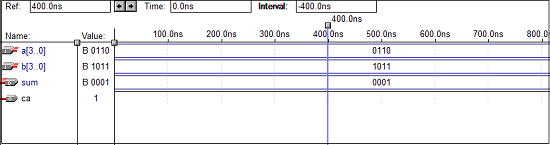## VHDL Code – 4 bit Parity Checker

library ieee;
use ieee.std_logic_1164.all;

entity parity_checker is
port (a0,a1,a2,a3 : in std_logic;
p : out std_logic);
end parity_checker;

architecture vcgandhi of parity_checker is
begin
p <= (((a0 xor a1) xor a2) xor a3);
end vcgandhi;


### Waveforms## VHDL Code – 4 bit Parity Generator

library ieee;
use ieee.std_logic_1164.all;

entity paritygen is
port (a0, a1, a2, a3: in std_logic; p_odd, p_even: out std_logic);
end paritygen;

architecture vcgandhi of paritygen is
begin
process (a0, a1, a2, a3)

if (a0 ='0' and a1 ='0' and a2 ='0' and a3 =’0’)
then odd_out <= "0";
even_out <= "0";
else
p_odd <= (((a0 xor a1) xor a2) xor a3);
p_even <= not(((a0 xor a1) xor a2) xor a3);
end vcgandhi


### Waveforms